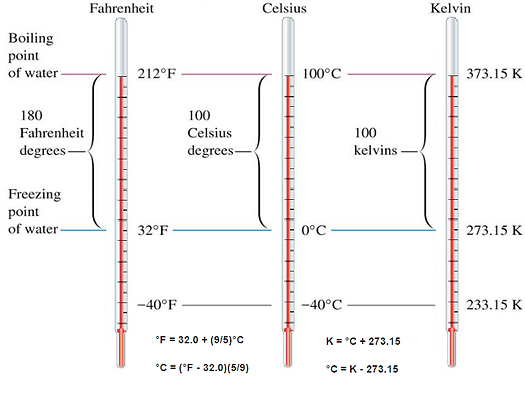Welcome to the Space Studies Chalk Board

Below, you will find many useful numbers and formulae utilized by aerospace and astronomy scientists. Some will include an external link, so the user may investigate the origin of individual formulae, scales, or tables.

Astronomical Distances:

Astronomical Unit (AU)= 1.496 x 10^8 km

Light Year (LY)≈ 9.46 x 10^12 km

Parsec (pc)≈ 3.09 x 10^13 km

Average distance to Moon≈ 238,900 mi

≈ 384,400 km

≈ 30 ErAstronomical/physical constants:

Speed of Light (C) = 299,792 km/s (in a vacuum)

= 186,282 mph

≈ 3.00 x 10^5 km/s

Gravitational Constant (G) = 6.674  x 10^-11 m^3/(kg s^2)

Planck's Constant (h) = 6.26 x 10^-34 Js

Stefan Boltzman Constant (σ) = 5.670 x 10^-8 W/m^2K^4

Proton Mass = 1.673 x 10^-27 kg

Electron Mass = 9.11 x 10^-31 kg

Solar Mass (Ms) = 2 x 10^30 kg

Radius of Sun = 696,000 km

Luminosity of Sun = 3.8 x 10^26 W

Mass of Earth = 5.97 x 10^24 kg

Temperature Scales and Conversions:

Credit to thermal-engineering.org